Test: Phase Modulation

# Test: Phase Modulation - Electronics and Communication Engineering (ECE)

Test Description

## 10 Questions MCQ Test GATE ECE (Electronics) 2023 Mock Test Series - Test: Phase Modulation

Test: Phase Modulation for Electronics and Communication Engineering (ECE) 2023 is part of GATE ECE (Electronics) 2023 Mock Test Series preparation. The Test: Phase Modulation questions and answers have been prepared according to the Electronics and Communication Engineering (ECE) exam syllabus.The Test: Phase Modulation MCQs are made for Electronics and Communication Engineering (ECE) 2023 Exam. Find important definitions, questions, notes, meanings, examples, exercises, MCQs and online tests for Test: Phase Modulation below.
Solutions of Test: Phase Modulation questions in English are available as part of our GATE ECE (Electronics) 2023 Mock Test Series for Electronics and Communication Engineering (ECE) & Test: Phase Modulation solutions in Hindi for GATE ECE (Electronics) 2023 Mock Test Series course. Download more important topics, notes, lectures and mock test series for Electronics and Communication Engineering (ECE) Exam by signing up for free. Attempt Test: Phase Modulation | 10 questions in 30 minutes | Mock test for Electronics and Communication Engineering (ECE) preparation | Free important questions MCQ to study GATE ECE (Electronics) 2023 Mock Test Series for Electronics and Communication Engineering (ECE) Exam | Download free PDF with solutions
 1 Crore+ students have signed up on EduRev. Have you?
Test: Phase Modulation - Question 1

### Which of the following phase modulation applications is INCORRECT?

Detailed Solution for Test: Phase Modulation - Question 1

A digital synthesizer is a synthesizer that uses digital signal processing (DSP) techniques to make musical sounds.

• Phase modulation is widely used for transmitting radio waves and is an integral element of many digital transmission coding schemes that support an ample range of wireless technologies such as GSM and Wi-Fi.
• It is also used in satellite television.
• Phase modulation is used in digital synthesizers for generating waveform and signal and not for transferring signals. (option 3 is incorrect)
• Phase modulation is very useful in radio waves transmission.
Test: Phase Modulation - Question 2

### In the phase modulated signal, the deviation of frequency is ________.

Detailed Solution for Test: Phase Modulation - Question 2

The frequency deviation in a phase-modulated carrier is proportional to the amplitude and frequency of the modulating signal.

Derivation:
A general expression for a phase-modulated wave is:
xPM (t) = A cos [2πfct + kpm(t)]
The instantaneous angle is given as:
ϕi(t) = 2πfct + kp m(t)
The instantaneous frequency (in Hz) will be obtained as: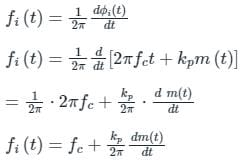Frequency deviation is given by: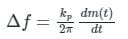For a single tone modulation:
m(t) = Am sin ωmt
The frequency deviation becomes: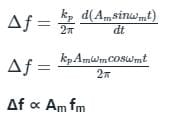Test: Phase Modulation - Question 3

### v = A sin (ωct + m sin ωmt) is the expression for

Detailed Solution for Test: Phase Modulation - Question 3

Analysis:
Let the carries signal Sc(t) = Ac sin ωct
The message signal m(t) = Am sin ωm t
So, the expression of FM is: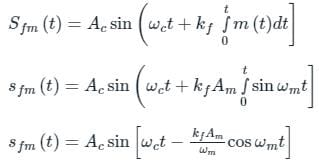If m: modulation index then: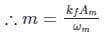Sfm(t) = Ac sin [ωct – m cos ωm t]
So hence given expression is not FM signal.
Now,
The expression of PM is:
Spm(t) = Ac sin (ωct + kp m(t)]
= Ac sin (ωc t + kp Am sin ωm t)
If m is the modulation index, then:
Spm(t) = Ac sin (ωc t + m sin ωm t)
∴ m = kp Am
Hence given expression is of PM signal.

Test: Phase Modulation - Question 4

Phase modulation is generally not used for transmission because

Detailed Solution for Test: Phase Modulation - Question 4
• Phase modulation (PM) is a modulation pattern that encodes information as variations in the instantaneous phase of a carrier wave
• Phase modulation is widely used for transmitting radio waves and is an integral part of many digital transmission coding schemes that underlie a wide range of technologies like Wi-Fi, GSM and satellite television
• Phase Modulation is generally not used for transmission because it is complex for decoding
Test: Phase Modulation - Question 5

In which of the following, the phase of the carrier wave changes in accordance with the intensity of light.

Detailed Solution for Test: Phase Modulation - Question 5

A wave has 3 parameters Amplitude, Phase, and Frequency. Thus there are 3 types of modulation techniques.

• Amplitude Modulation: The amplitude of the carrier is varied according to the amplitude of the message signal or the intensity of light.
• Frequency Modulation: The frequency of the carrier is varied according to the amplitude of the message signal or the intensity of light.
• Phase Modulation: The Phase of the carrier is varied according to the amplitude of the message signal or the intensity of light.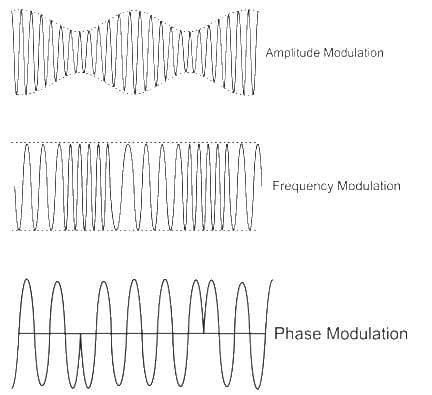*Answer can only contain numeric values
Test: Phase Modulation - Question 6

An angle modulated signal with carrier frequency ωc = 2π × 106 rad/s given by ϕm(t) = cos(ωct + 5 sin (1000πt) + 10 sin (2000πt)). The maximum deviation of the frequency in the angle modulated signal from that of the carrier is ___________ kHz.

Detailed Solution for Test: Phase Modulation - Question 6

Given:
The angle modulated signal is given by
ϕm(t) = cos(ωct + 5 sin (1000πt) + 10 sin (2000πt))
instantaneous frequency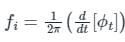Where ϕt is the phase of angle modulated signal.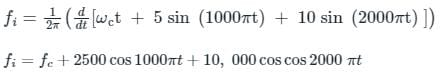In the above expression
fc is the carrier frequency and additional term is the deviation (2500 cos 1000πt + 10, 000 cos cos 2000 πt)
fd = 2500 cos 1000πt + 10, 000 cos cos 2000 πt
(fD)max = max (2500 cos 1000πt + 10, 000 cos cos 2000 πt)
(fD)max = (2500 + 10, 000)Hz = 12.5 kHz

Test: Phase Modulation - Question 7

A phase modulated signal can be obtained from frequency modulator by passing the modulating signal through a

Detailed Solution for Test: Phase Modulation - Question 7

The output of FM modulator is of form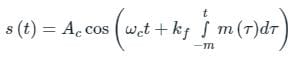After passing the modulating signal through the differentiator we give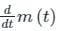as the input to the frequency modulator, i.e.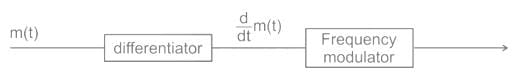The expression of the output signal will be: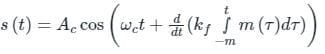The differentiation of the integral will simply give the original signal.
We get a phase modulated signal which is of the form: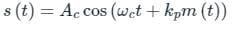Test: Phase Modulation - Question 8

In phase modulated signal, the frequency deviation is proportional to

Detailed Solution for Test: Phase Modulation - Question 8

The frequency deviation in a phase-modulated carrier is proportional to the amplitude and frequency of the modulating signal both.
Derivation:
A general expression for a phase-modulated wave is:
xPM (t) = A cos [2πfct + kpm(t)]
The instantaneous angle is given as:
ϕi(t) = 2πfct + kp m(t)
The instantaneous frequency (in Hz) will be obtained as: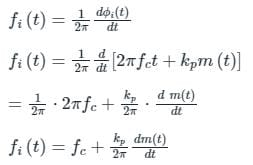Frequency deviation is given by: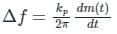For a single tone modulation:
m(t) = Am sin ωmt
The frequency deviation becomes: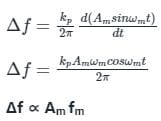Test: Phase Modulation - Question 9

A message signal m(t) = Am sin (2πfmt) is used to modulate the phase of a carrier Ac cos (2πfct) to get the modulated signal y(t) = Ac cos (2πfct + m(t)). The bandwidth of y(t)

Detailed Solution for Test: Phase Modulation - Question 9

Concept:
If m(t) is the message single & c(t) = Ac cos(ωct) is the Carrier signal, then the General expression for a Phase modulated signal is:
SPM(t) = Ac cos[ωct + kpm(t)]
Where kp = Phase Sensitivity (rad/Volts)

Analysis:
SPM(t) = Accos[ωct + kpm(t)] = Ac cos(θi)
Instantaneous Phase θi(t) = ωc(t) + kpm(t)
Instantaneous frequency ωi(t) =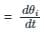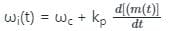• Phase deviation Δϕ = kp m(t)
• Frequency deviation Δω =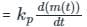• Deviation ratio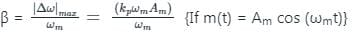According to Carson formula, the Bandwidth of a phase-modulated signal is:
BW = 2(β + 1) ωm = 2(kpAm + 1)ωm
Hence the Bandwidth depends on both Am & ωm
So, Option (3) is Correct.

*Answer can only contain numeric values
Test: Phase Modulation - Question 10

The baseband signal m(t) shown in the figure is phase-modulated to generate the PM signal φ(t) = cos(2πfct + km(t)). The time t on the x-axis in the figure is in milliseconds. If the carrier frequency fc = 50 kHz and k = 10π, then the ratio of the minimum instantaneous frequency (in kHz) to the maximum instantaneous frequency (in kHz) is __________ (rounded off to 2 decimal places).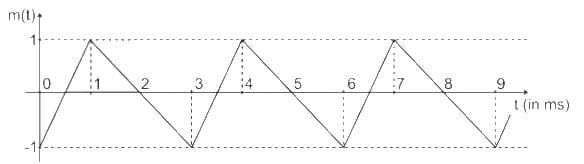Detailed Solution for Test: Phase Modulation - Question 10

Concept:
The instantaneous angular frequency will be obtained by differentiating the instantaneous phase as: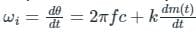if θ (t) = 2π fct + k m(t)

Calculation:
Given Φ(t) = cos (2π fct + km(t))
fc = 50 kHz
K = 10 π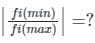Phase of the modulated signal is given by:
θ (t) = 2π fct + k m(t)
The instantaneous angular frequency will be obtained by differentiating the instantaneous phase as: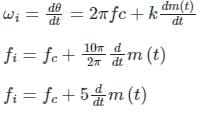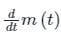is the slope of the message signal:
maximum positive slope (between 0 ≤ t ≤ 1) is 2
Maximum negative slope (between +1 < t < 3) is:
= -2/2 = -1
fi max = 50 + 5(2) = 60 kHz
fi min = 50 – 5(1) = 45 kHz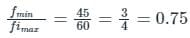## GATE ECE (Electronics) 2023 Mock Test Series

21 docs|263 tests
Information about Test: Phase Modulation Page
In this test you can find the Exam questions for Test: Phase Modulation solved & explained in the simplest way possible. Besides giving Questions and answers for Test: Phase Modulation, EduRev gives you an ample number of Online tests for practice

## GATE ECE (Electronics) 2023 Mock Test Series

21 docs|263 tests(Scan QR code)